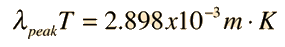To find the peak of the radiation curve as indicated in Wien's displacement law, it is necessary to take the derivative of the Planck radiation formula with respect to wavelength. Setting this derivative equal to zero to determine the maximum gives the equationShow

This is a trancendental equation which must be solved numerically. It gives the result:Calculation

Index

 HyperPhysics***** Quantum Physics R Nave
Go Back

/Users/RodNave/Documents/HyperPhysics/hbase/quantum/imgqua/wiend5.png

# Finding the Blackbody Peak

 From the Planck radiation formulathe evaluation of the maximum yields Wien's displacement law To find the peak of the blackbody radiation curve, we take the derivative:Simplifying gives the maximum condition:which must be solved numerically to give:Index